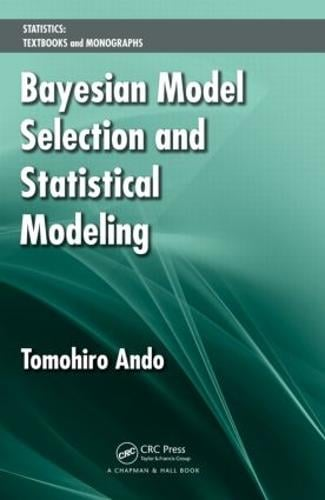•Bayesian Model Selection and Statistical Modeling (Hardback)

(author)
£82.99
Hardback 300 Pages / Published: 27/05/2010
• We can order this

Usually dispatched within 3 weeks

Along with many practical applications, Bayesian Model Selection and Statistical Modeling presents an array of Bayesian inference and model selection procedures. It thoroughly explains the concepts, illustrates the derivations of various Bayesian model selection criteria through examples, and provides R code for implementation.

The author shows how to implement a variety of Bayesian inference using R and sampling methods, such as Markov chain Monte Carlo. He covers the different types of simulation-based Bayesian model selection criteria, including the numerical calculation of Bayes factors, the Bayesian predictive information criterion, and the deviance information criterion. He also provides a theoretical basis for the analysis of these criteria. In addition, the author discusses how Bayesian model averaging can simultaneously treat both model and parameter uncertainties.

Selecting and constructing the appropriate statistical model significantly affect the quality of results in decision making, forecasting, stochastic structure explorations, and other problems. Helping you choose the right Bayesian model, this book focuses on the framework for Bayesian model selection and includes practical examples of model selection criteria.

Publisher: Taylor & Francis Ltd
ISBN: 9781439836149
Number of pages: 300
Weight: 544 g
Dimensions: 235 x 159 x 18 mm